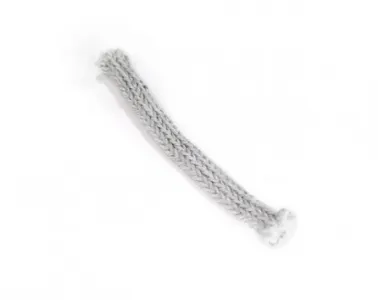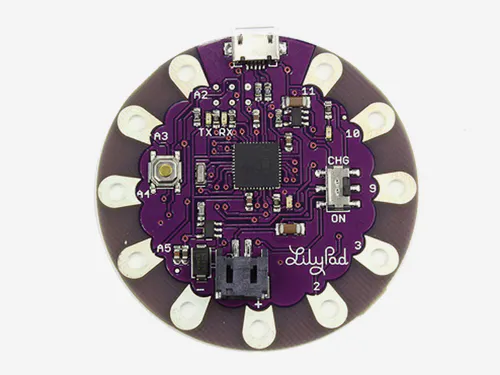# Tubolar Stretch Sensor Tutorial © GPL3+

How to read the value of a Tubolar Stretch Sensor with your Arduino.

• 5,658 views
• 7 respects

## Components and supplies×1
 Tubolar Stretch Sensor (10cm peace)
×1
 10 1k 1/4w resistor
×1

### Connect the sensor to the power supply

The Tubolar Stretch Sensor is an analog textile sensor that changes its resistivity when it is pulled and stretched. It is useful for creating variable voltage divider that can be easily read by an Arduino.

### Connect the input to the sensor

Connect one end of the sensor to the 5V pin of the Lilypad. Then you need to connect the other end to ground through a 1 kOhm resistor like shown in the picture.

### How to read values with the Arduino

Now connect the analog input pin A5 of the Arduino to the end of the sensor connected to the resistor.

### How to read the sensor value with Arduino

The sensor changes its resistance if you stretch it. This will change the voltage read by the Arduino.

``````int sensorPin = A5;    // select the input pin for the sensor
int ledPin = 13;      // select the pin for the LED
int sensorValue = 0;  // variable to store the value coming from the sensor

void setup() {
// declare the ledPin as an OUTPUT:
pinMode(ledPin, OUTPUT);
}

void loop() {
// read the value from the sensor:
// turn the ledPin on
digitalWrite(ledPin, HIGH);
// stop the program for <sensorValue> milliseconds:
delay(sensorValue);
// turn the ledPin off:
digitalWrite(ledPin, LOW);
// stop the program for for <sensorValue> milliseconds:
delay(sensorValue);
}
``````

## Code

##### Code snippet #1Arduino
```int sensorPin = A5;    // select the input pin for the sensor
int ledPin = 13;      // select the pin for the LED
int sensorValue = 0;  // variable to store the value coming from the sensor

void setup() {
// declare the ledPin as an OUTPUT:
pinMode(ledPin, OUTPUT);
}

void loop() {
// read the value from the sensor:
// turn the ledPin on
digitalWrite(ledPin, HIGH);
// stop the program for <sensorValue> milliseconds:
delay(sensorValue);
// turn the ledPin off:
digitalWrite(ledPin, LOW);
// stop the program for for <sensorValue> milliseconds:
delay(sensorValue);
}
```

July 8, 2016

#### Members who respect this project

See similar projects
you might like

#### How to Make FLEX Sensor at Home | DIY Flex Sensor

Project tutorial by Tech Nuttiez

• 1,394 views
• 1 comment
• 10 respects

#### Analog Textile Press Button Tutorial

Project tutorial by Arduino_Scuola

• 3,236 views
• 8 respects

• 38,681 views
• 45 respects

• 18,645 views
• 22 respects

#### Sitting Sensor

Project tutorial by kozlowaa

• 3,819 views